Math resources Geometry Area

Area of isosceles triangle

# Area of isosceles triangle

Here you will learn about how to find the area of isosceles triangles, including what formula to use and why it works.

Students will first learn about the area of isosceles triangles as part of geometry in 6 th grade.

## What is the area of an isosceles triangle?

The area of an isosceles triangle is the amount of the space inside an isosceles triangle.

An isosceles triangle is a type of triangle with two equal sides. The base angles, which are opposite to the sides of equal length, are also two equal angles. It always has one unequal side and angle.

In order to find the area of isosceles triangles, start with the area of a rectangle.

The area of the rectangle is calculated by multiplying the \text {base } \times \text { height}.

Within a rectangle, there is an isosceles triangle. It can be shown by drawing lines from a midpoint of the rectangle to the opposite vertices.

Creating the isosceles triangle (in pink) also creates two congruent right triangles (in green).

If you rotate the two right triangles and place them back to back, they form an isosceles triangle that is the same area as the pink isosceles triangle.

The area of the rectangle is the same as two congruent isosceles triangles. This means the area of each isosceles triangle is exactly half the area of the rectangle.

The general formula to find the area of any triangle is:

\text {Area of a triangle }=\cfrac{\text { base } \times \text { height }}{2}

This can also be written as the following formula:

A=\cfrac{1}{2} \, b h

where b is the base length and h is the height of the triangle.

The space inside of shapes is measured in square units, so the final answer must be given in \text {units}^2 . For example, \, \mathrm{cm}^2, \mathrm{~m}^2, or \mathrm{~mm}^2.

For example,

What is the area of the isosceles triangle?

A=\cfrac{1}{2} \, b h

The base is 10 and the height is 12 .

A few things to note:

• The height of an isosceles triangle is always a perpendicular line that starts at a vertex and intersects with the opposite side of the triangle at a 90 degree angle.
• The other side lengths of the triangle may be given, but they are extra information.

\begin{aligned} & A=\cfrac{1}{2} \, \times10 \times 12 \\\\ & A=5 \times 12 \\\\ & A=60 \end{aligned}

The area of the isosceles triangle is 60 {~cm}^2.

### What is the area of an isosceles triangle?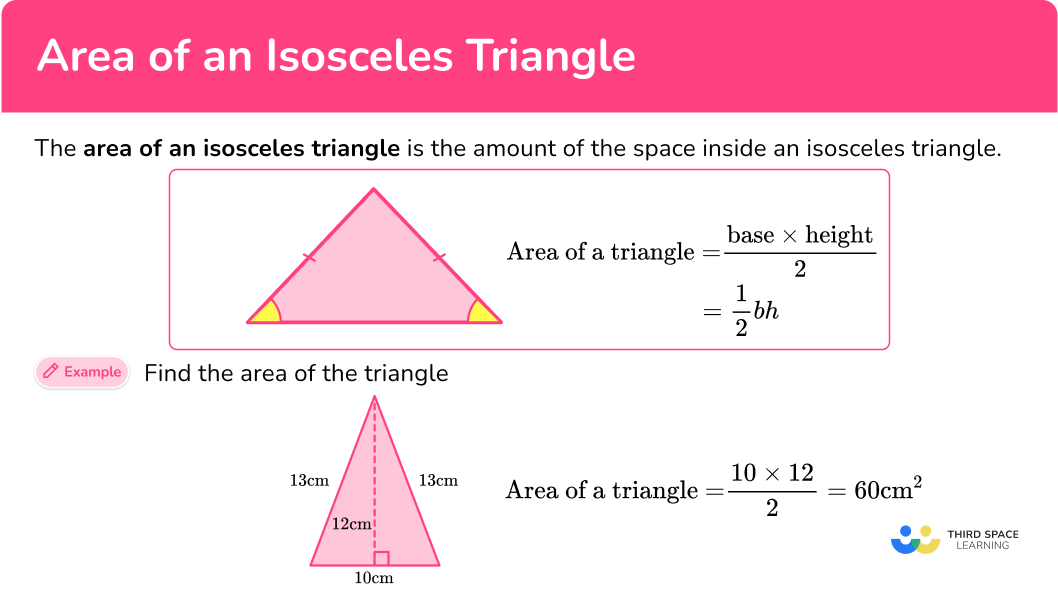## Common Core State Standards

How does this relate to 6 th grade math?

• Grade 6 – Geometry (6.G.A.1)
Find the area of right triangles, other triangles, special quadrilaterals, and polygons by composing into rectangles or decomposing into triangles and other shapes; apply these techniques in the context of solving real-world and mathematical problems.

## How to find the area of isosceles triangles

In order to find the area of isosceles triangles:

1. Identify the base and perpendicular height of the triangle.
2. Write the area formula.
3. Substitute known values into the area formula.
4. Solve the equation.
5. Write the answer, including the units.

## Area of isosceles triangles examples

### Example 1: given base length and height

Find the area of the triangle below:

1. Identify the base and perpendicular height of the triangle.

b = 6 {~cm}

h = 10 {~cm}

2Write the area formula.

A=\cfrac{1}{2} \, b h

3Substitute known values into the area formula.

\begin{aligned}A &=\cfrac{1}{2} \, b h \\\\ &=\cfrac{1}{2} \, (6)(10) \end{aligned}

4Solve the equation.

\begin{aligned}A &=\cfrac{1}{2} \, b h \\\\ &=\cfrac{1}{2} \, (6)(10) \\\\ &=30 \end{aligned}

5Write the answer, including the units.

A=30 \mathrm{~cm}^2

### Example 2: given base length and height

Calculate the area of the triangle below:

Rotating the triangle makes it easier to identify the base and height.

b = 4 {~m}

h = 120 {~cm}

Notice there are 2 different units here. You must convert them to a common unit:

120 {~cm} = 1.2 {~m}

b = 4 {~m}

h = 1.2 {~m}

A=\cfrac{1}{2} \, b h

\begin{aligned} A &=\cfrac{1}{2} \, b h \\\\ &=\cfrac{1}{2} \, (4)(1.2) \end{aligned}

\begin{aligned} A &=\cfrac{1}{2} \, b h \\\\ &=\cfrac{1}{2} \, (4)(1.2)\\\\ &=2.4 \end{aligned}

A=2.4 {~m}^2

### Example 3: word problem with 3 measurements given

Shown below is a triangular shaped field. Each cow needs 2,000 \mathrm{~m}^2 to graze. How many cows can fit into this field?

b = 312 {~m}

h = 137.6 {~m}

Note: The 208 {~m} is not needed to solve this question. It is extra information.

A=\cfrac{1}{2} \, b h

\begin{aligned} A & =\cfrac{1}{2} \, b h \\\\ & =\cfrac{1}{2} \, (312)(137.6) \end{aligned}

\begin{aligned} A & =\cfrac{1}{2} \, b h \\\\ & =\cfrac{1}{2} \, (312)(137.6) \\\\ & =21,465.6 \end{aligned}

A=21,465.6 \mathrm{~m}^2

Now to calculate how many cattle will fit into the field, take 21,465.6\mathrm{~m}^2 and divide it by 2,000\mathrm{~m}^2 , because each cattle needs that much space to graze on the field.

21,465.6 \div 2,000=10.7328

The quotient has 10 wholes and 7,328 ten-thousandths. There cannot be part of a cow and 10.7328 is not enough for 11 cows. So, 10 cows will fit in the field.

### Example 4: given half of base length, hypotenuse and height

Find the area of the triangle below:

Since one half of the base is 2 {~cm} , the other half is 2 {~cm} as well and 2 + 2 = 4.

b = 4 {~cm}

h = 6.71 {~cm}

A=\cfrac{1}{2} \, b h

\begin{aligned} A &=\cfrac{1}{2} \, b h \\\\ &=\cfrac{1}{2} \, (4)(6.71) \end{aligned}

\begin{aligned} A &=\cfrac{1}{2} \, b h \\\\ &=\cfrac{1}{2} \, (4)(6.71)\\\\ &=13.42 \end{aligned}

A=13.42 \mathrm{~cm}^2

### Example 5: compound shapes

Below is the floor plan for a new house. Calculate the area of the plan.

Split the plan into 2 shapes; a square and an isosceles triangle.

For the triangle:

b = 8 {~m}

h = 11 {~m}

A=\cfrac{1}{2} \, b h

\begin{aligned} A &=\cfrac{1}{2} \, b h \\\\ &=\cfrac{1}{2} \, (8)(11) \end{aligned}

\begin{aligned} A &=\cfrac{1}{2} \, b h \\\\ &=\cfrac{1}{2} \, (8)(11)\\\\ &=44 \end{aligned}

Now find the area of the square.

\begin{aligned} & A=s^2 \\\\ & A=8^2 \\\\ & A=64 \end{aligned}

The total area of the compound shape is 44+64=108 {~m}^2.

### Example 6: calculating base length

Triangle ABC is an isosceles triangle with an area of 18 {~cm}^2 . The height of the triangle is 6 {~cm} . Find the length of the base of the triangle.

\begin{aligned} & A=18 \mathrm{~cm}^2 \\\\ & b=? \\\\ & h=6 \mathrm{~cm} \end{aligned}

A=\cfrac{1}{2} \, b h

\begin{aligned} & A=\cfrac{1}{2} \, b h \\\\ & 18=\cfrac{1}{2} \times b \times 6 \\\\ & 18=\cfrac{1}{2} \times 6 \times b \\\\ & 18=3 \times b \end{aligned}

18=3 \times b

To solve, find what times 3 is equal to 18. Since 3 \times 6=18 , the base is 6.

b=6 \mathrm{~cm}

The units are not squared, because the base is the length of the bottom of the triangle (one-dimensional), not the square units within the triangle (two-dimensional).

### Teaching tips for area of isosceles triangles

• In the beginning, prioritize activities that connect the area of a rectangle to the area of a triangle. For example, on gridded paper have students find the area of various rectangles and the isosceles triangles within them. They will begin to notice that the area of the triangles is half the area of a rectangle.

Note that because students will have to add partial square units together, their answers may not be exactly half. However, this is not a reason to avoid the activity, but instead it provides an opportunity to discuss why their answers may vary slightly and why a formula provides more precise answers.

• Be sure to expose students to all types of isosceles triangles (acute, right and obtuse) and in all different orientations. It is important that students become comfortable identifying the base and the height no matter what type of triangle or its position. Always look for worksheets or study materials that provide this type of variety.

### Easy mistakes to make

• Using the wrong measurement as the base or the height
A question may give extra information that is not needed to answer it. Carefully identify the base and the height of a triangle by looking for the perpendicular relationship between them.
For example,
To calculate the area, only use the base and height.

Base= 12 {~cm}
Height = 8 {~cm}
Area = \cfrac{12 \, \times \, 8}{2} = 48 {~cm}^{2}

• Forgetting to write the units
It is common to forget the units for area in the final answer. When calculating the area, the answer must always have units squared.

• Writing the incorrect units
Pay close attention to what measurement is being recorded. When solving for a missing base or height length using the area, the answer will be recorded in units, not square units. It is also common to confuse area units with volume units, once the topic is introduced.

This is why activities that include grids for area and cubed units for volume are important to integrate throughout the learning of these topics.

### Practice area of isosceles triangles questions

1. Find the area of the triangle below: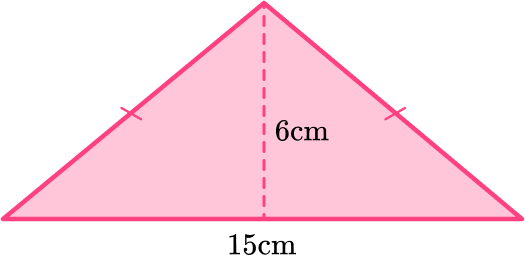90 {~cm}^{2}45 {~cm}^{2}180 {~cm}^{2}21 {~cm}^{2}The area of a triangle is calculated with the formula: A=\cfrac{1}{2} \, b h.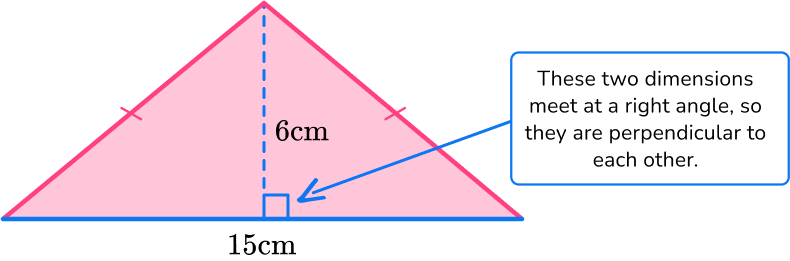For this triangle:

b = 15 {~cm}

h = 6 {~cm}

Substitute the values into the formula.

\begin{aligned} A & =\cfrac{1}{2} \, b h \\\\ & =\cfrac{1}{2} \, (15)(6) \\\\ & =45 \mathrm{~cm}^2 \end{aligned}

2. Find the area of the triangle below giving your answer in cm^2 :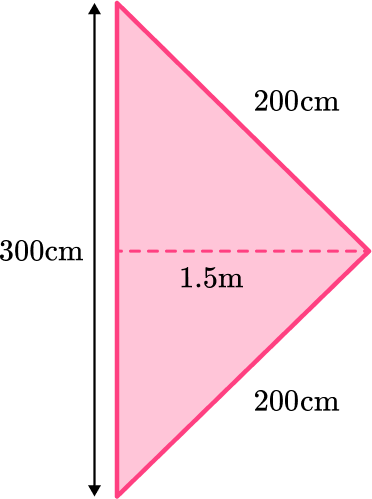225 \mathrm{~cm}^22,250 \mathrm{~cm}^222,500 \mathrm{~cm}^245,000 \mathrm{~cm}^2The area of a triangle is calculated with the formula: A=\cfrac{1}{2} \, b h.

Rotating the triangle makes it easier to identify the base and height.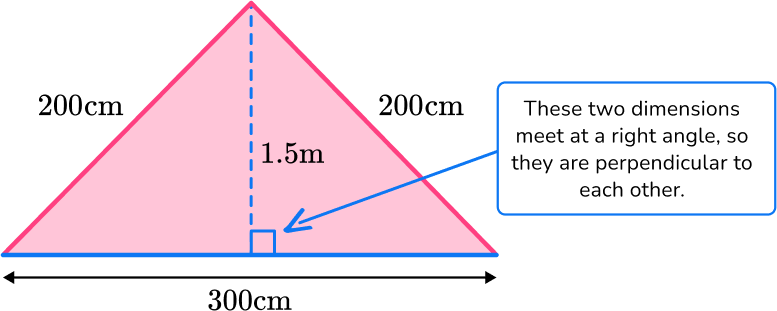For this triangle:

b = 300 {~cm}

h = 1.5 {~m}

Since the measurements are in different units (m and cm), they need to be converted to the same unit:  1.5 {~m} = 150 {~cm}.

b = 300 {~cm}

h = 150 {~cm}

Note: 200 \, cm is not needed to solve this question. It is extra information.

Substitute the values into the formula.

\begin{aligned} A & =\cfrac{1}{2} \, b h \\\\ & =\cfrac{1}{2} \, (300)(150) \\\\ & =22,500 \mathrm{~cm}^2 \end{aligned}

3. Shown below is a triangular shaped chicken enclosure cage. Each chicken needs 8 {~m}^2 to roam around. How many chickens can fit into this cage?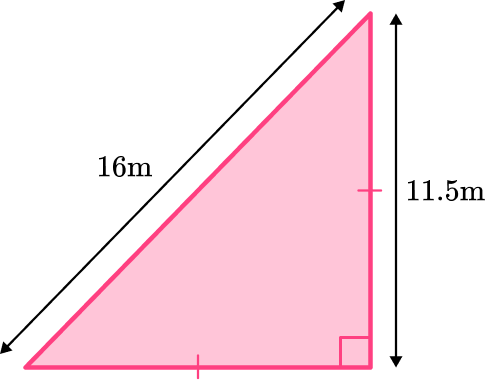8 chickens11 chickens66 chickens9 chickensThe area of a triangle is calculated with the formula: A=\cfrac{1}{2} \, b h.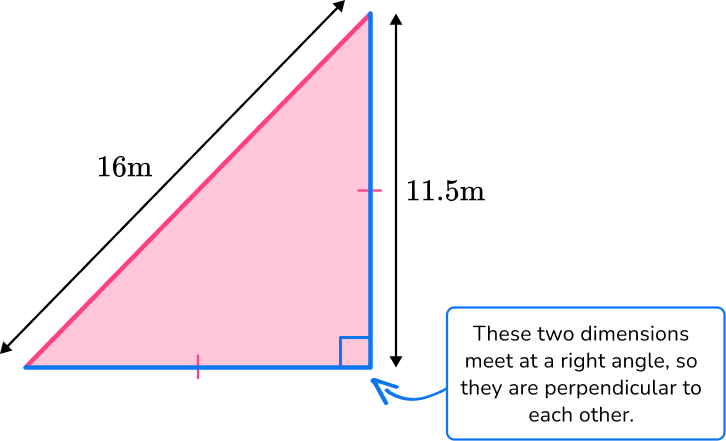This is an isosceles right triangle, so the base and the height are equal to each other.

For this triangle:

b = 11.5 {~m}

h = 11.5 {~m}

Note: The 16 {~m} is not needed to solve this question. It is extra information.

\begin{aligned} A & =\cfrac{1}{2} \, b h \\\\ & =\cfrac{1}{2} \, (11.5)(11.5) \\\\ & =66.125 m^2 \end{aligned}

Now to calculate how many chickens will fit into the field, take 66.125 {~m}^2 and divide it by 8 {~m}^2 because each chicken needs that much space to graze on the field.

66.125 \div 8=8.2656

The quotient has 8 wholes and 2,656 ten-thousandths. There cannot be part of a chicken and 8.2656 is not enough for 9 chickens. So, 8 chickens will fit in the field.

4. Find the area of the triangle below. Round to the nearest hundredth.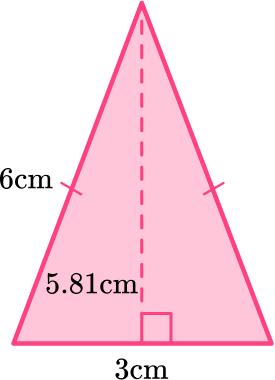18.00 {~cm}^{2}17.42 {~cm}^{2}9.00 {~cm}^{2}8.72 {~cm}^{2}The area of a triangle is calculated with the formula: A=\cfrac{1}{2} \, b h.

Remember, the height and base are always perpendicular to each other.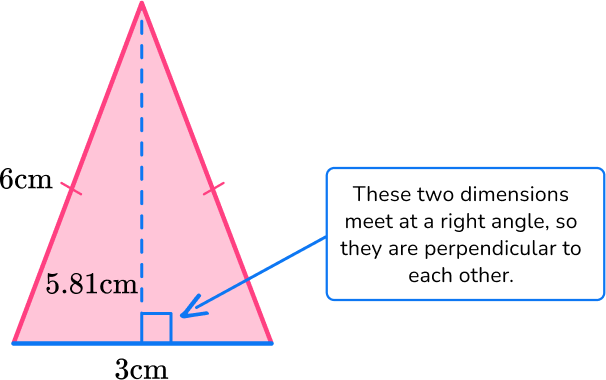b = 3{~cm}

h = 5.81{~cm}

Note: The 6 {~cm} is not needed to solve this question. It is extra information.

\begin{aligned} A & =\cfrac{1}{2} \, b h \\\\ & =\cfrac{1}{2} \, (3)(5.81) \\\\ & =8.715 \mathrm{~cm}^2 \end{aligned}

8.715c{~cm}^2 rounded to the nearest hundredth is 8.72 {~cm}^2.

5. Find the area of the shaded region below. Round to the nearest tenth.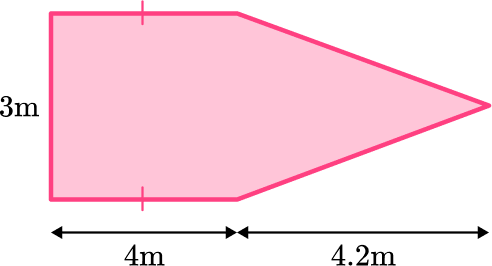24.6 {~m}^{2}18.3 {~m}^{2}12.3 {~m}^{2}19.4 {~m}^{2}The shape can be split into a rectangle and a triangle. The opposite sides of the rectangle are congruent.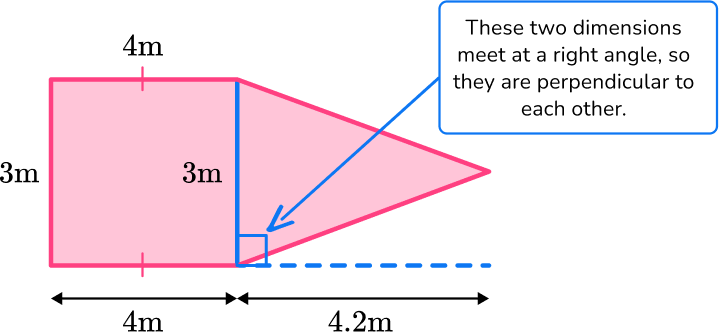The area of the rectangle is  3 \times 4 = 12 {~m}^{2}

The area of the triangle is \cfrac{1}{2} \, \times 3 \times 4.2 = 6.3 {~m}^{2}

The total area is 12 + 6.3 = 18.3 {~m}^{2}

6. Triangle MNP is an isosceles triangle with an area of 20 {~cm}^2 . The base length of the triangle is 4 {~cm} . Find the height of the triangle.

80 {~cm}5 {~cm}2.5 {~cm}10 {~cm}The area of a triangle is calculated with the formula: A=\cfrac{1}{2} \, b h.

\begin{aligned} & A=20 \mathrm{~cm}^2 \\\\ & b=? \\\\ & h=4 \mathrm{~cm} \end{aligned}

\begin{aligned} & A=\cfrac{1}{2} \, b h \\\\ & 20=\cfrac{1}{2} \,\times b \times 4 \\\\ & 20=\cfrac{1}{2} \, \times 4 \times b \\\\ & 20=2 \times b \end{aligned}

To solve, find what times 2 is equal to 20 . Since 2 \times 10=20 , the base is 10 .

## Area of isosceles triangles FAQs

Where is the base of the isosceles triangle?

The base is considered the side adjacent to the two congruent sides. However, to find the area any side of the triangle can be used as the base. In order to be used as the base measurement, the height measurement should go directly up from the base, forming a 90 degree angle.

How do you find the perimeter of an isosceles triangle?

To find the perimeter, add up all three sides of an isosceles triangle.

What is the pythagorean theorem?

The pythagorean theorem explains a relationship between the sides of all right triangles. It is used in trigonometry to find a missing third side measurement.

What is the hypotenuse?

The hypotenuse is the longest side of a right triangle. It is opposite of the vertex angle measuring 90 degrees.

## Still stuck?

At Third Space Learning, we specialize in helping teachers and school leaders to provide personalized math support for more of their students through high-quality, online one-on-one math tutoring delivered by subject experts.

Each week, our tutors support thousands of students who are at risk of not meeting their grade-level expectations, and help accelerate their progress and boost their confidence.